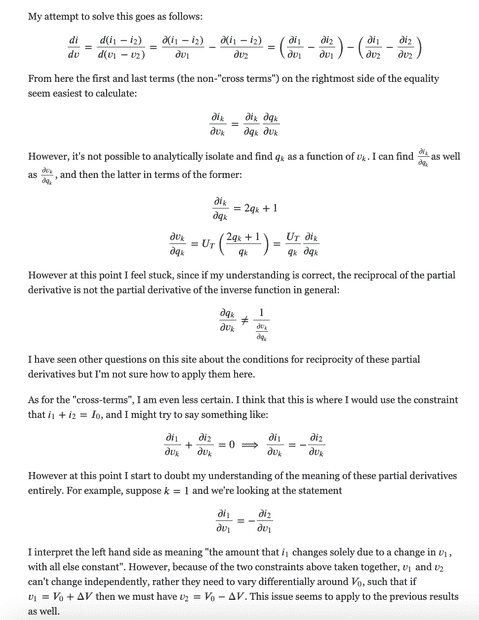# Calculating total derivative of multivariable function

• halleffect

#### halleffect

Homework Statement
Here is the full problem statement (with equations): https://i.imgur.com/z2SWodC.png The answer that I'm trying to derive is at the bottom.
Relevant Equations
All equations are also included in the above image (https://i.imgur.com/z2SWodC.png).
This isn't a homework problem exactly but my attempt to derive a result given in a textbook for myself. Below is my attempt at a solution, typed up elsewhere with nice formatting so didn't want to redo it all. Direct image link here. Would greatly appreciate if anyone has any pointers.#### Attachments

Last edited by a moderator:
By ##I_0## and ##V_0## sum conditons, we know
$$i=2i_1-I_0=i(q_1)$$
$$v=2v_1-V_0=v(q_1)$$
They both are functions of variable ##q_1## only.
$$\frac{di}{dv}=\frac{\frac{di}{dq_1}} {\frac{dv}{dq_1}}=\frac{\frac{di_1}{dq_1}} {\frac{dv_1}{dq_1}}$$
we can calculate it.

Last edited:
•topsquark
By ##I_0## and ##V_0## sum conditons, we know
$$i=2i_1-I_0=i(q_1)$$
$$v=2v_1-V_0=v(q_1)$$
They both are functions of variable ##q_1## only.
$$\frac{di}{dv}=\frac{\frac{di}{dq_1}} {\frac{dv}{dq_1}}=\frac{\frac{di_1}{dq_1}} {\frac{dv_1}{dq_1}}$$
we can calculate it.

Thanks very much for the reply; with that info:

$$\frac{di_1}{dq_1} = 2q_1+1$$
$$\frac{dv_1}{dq_1} = U_T(\frac{2q_1+1}{q_1})$$

And from this I get

$$\frac{di}{dv}=\frac{ \frac{di_1}{dq_1} }{ \frac{dv_1}{dq_1} }= \frac{q_1}{U_T}$$

I'm not sure if this is correct. For one thing, I'm not sure how I could incorporate ##q_2## into the answer like the given one (which is ##\frac{2}{U_T}\frac{q_1 q_2}{q_1+q_2}##), although it does seem to make sense that it should be expressible through just one of the ##q##'s. Is there a way to show this to be equal to the given answer? (Assuming I haven't made an error here)